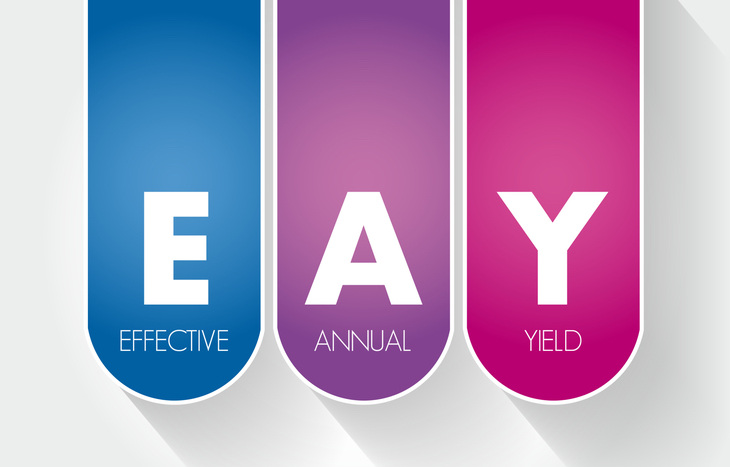Home Financial Literacy What is Effective Yield?

# What is Effective Yield?

When buying a bond, yield is one of the most important factors to consider. But calculating yield can vary depending on what the investor does with their coupon payments. Will you pocket them as a form of income? Or, will you reinvest those payments at the same rate? If you choose the latter, you’ll want to calculate the bond’s effective yield.

Effective yield represents the total return on a bond assuming the bondholder reinvests each coupon payment at the same rate. As you might imagine, this measure of yield is higher than the nominal yield of the bond, which assumes no reinvestment. Effective yield recognizes the compounding power of interest payments, and it’s an important measure of the worth of long-term bonds.

Here’s a closer look at effective yield, including how to calculate it, what it represents and how it stacks up to other bond valuation metrics.## How to Calculate Effective Yield

Also called the “effective rate of interest,” effective yield looks closely at the compounding power of interest over the term of a bond. To calculate this rate, investors need to annualize the compounding power of reinvested coupon payments. The formula looks like this:

Effective Yield = [1 + (r/n)]n – 1

In the above equation, “r” represents the bond’s coupon rate and “n” represents the number of payments per year. As investors plug in the relevant data about a bond, they’ll begin to see the power of compound interest rate shape. Here’s an example:

Jim wants to invest in a 10-year, \$1,000 bond with a 6% coupon rate. The bond pays interest quarterly (four per year). Jim can expect four \$15 payments, amounting to \$60 total. However, he plans to reinvest these payments. He calculates the effective yield of the bond at [1 + (0.06/4)4 – 1, which amounts to 6.13%.

The example above assumes that, all factors consistent, Jim’s reinvested coupon payments will net him a higher yield than had he taken them as payments outright. The more coupon payments, the high the effective yield becomes relative to the interest rate.

## What is Nominal Yield?

The two most basic metrics bond investors will look at when evaluating bond ROI are effective yield and nominal yield. They’re not the only methods for looking at bond value over the term of the asset, but they are the most straightforward. We’ve covered the effective yield above. So what is nominal yield?

Nominal yield is essentially the bond’s coupon rate. For instance, if the bond has a par value of \$1,000 and a coupon rate of 5%, the bond’s nominal yield is 5% or \$50. Keep in mind that this figure and its behavior can change depending on the bond. Fixed bonds will have a standard nominal yield, while the nominal yield of floating and indexed bonds will change over the bond’s term.

The chief difference between these two measures is the accounting of interest. If you plan to reinvest coupon payments, effective yield provides an accurate ROI. If the bond serves as a means to passive income, you won’t reinvest and thus, won’t compound the yield.

## The Drawbacks of Effective Yield as a Measure

There are a couple of important considerations to keep in mind when looking at effective yield.

First, it assumes that bondholders reinvest coupon payments in another vehicle that pays exactly the same rate as the bond. Typically, this is another fixed-rate investment. If, for some reason, the investor puts their coupon payments into an investment with a fluctuating interest rate, it’ll invalidate the effective rate of interest.

The other often-overlooked problem with this measurement is that it assumes investors acquired their bond at its par value, not a discount or premium. This can skew the measure of yield, which is why it’s also important to calculate the bond’s yield to maturity (YTM).

## Here’s Why Yield to Maturity is Important

Investors calculating effective yield will also often calculate YTM, then compare the two through the lens of effective annual yield. Effective yield measures a single year; effective annual yield annualized this figure to make it comparable across the term of the bond.

YTM serves as the bond equivalent yield. Having the bond equivalent yield allows investors to more accurately calculate a bond’s total ROI because it factors in the time value of money. Juxtaposed next to each other, the figures help investors understand whether the bond trades at a premium or a discount, and what type of yield they can expect from it.

• If the bond’s YTM is higher than its effective yield, it’ll trade at a discount
• If the bond’s YTM is lower than its effective yield, it’ll trade at a premium

## Looking Closer at the True Yield of a Bond

When evaluating the potential ROI on a long-term bond, remember that the stated interest rate of the bond isn’t necessarily indicative of its yield. Calculating the bond’s effective yield provides a more accurate insight into what an investor can expect to see in terms of return. Extrapolated over the life of the bond, there’s an opportunity for investors to better-understand the effects of compounding over the entire bond term.

For investors who simply want fixed income payments, this isn’t necessarily something to worry about. No reinvestment means no need to compound the yield. However, for bond traders and those trying to evaluate compounding effects, effective yield is useful to know.